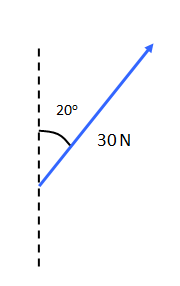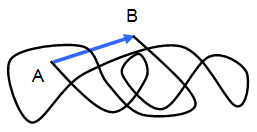# Scalars and vectors

The quantities measured in Physics may be divided into two groups:

(a) scalars - these are quantities that have magnitude (size) only.

Examples of scalars are length, speed, mass, density, energy, power, temperature, charge, potential difference

(b) vectors - these are quantities that have direction as well as magnitude.
Examples of vectors are displacement, force, torque, velocity, acceleration, momentum, electric current, magnetic flux density, electric fieldScalars may be added together by simple arithmetic but when two or more vectors are added together their direction must be taken into account as well.

A vector may be represented by a line, the length of the line being the magnitude of the vector and the direction of the line the direction of the vector.

For example Figure 1 shows a force of 30 N acting at 20o to the vertical of the page.A simple comparison between a vector and a scalar is shown by Figure 2. This shows the distance moved by a soccer referee during the match. The vector represents the displacement between the starting position (A) and his position at the end of the game (B) while the wavy line (a scalar) is the distance that the referee has actually run during the game (much further!).

When it is said that a vector has a direction it means that either it is moving in a certain direction (as in a velocity) or that it could produce movement in a certain direction (as in a force or a magnetic field).

A VERSION IN WORD IS AVAILABLE ON THE SCHOOLPHYSICS USB